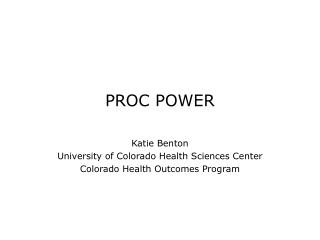DownloadDownload PresentationPROC POWER

# PROC POWER

Télécharger la présentation## PROC POWER

- - - - - - - - - - - - - - - - - - - - - - - - - - - E N D - - - - - - - - - - - - - - - - - - - - - - - - - - -
##### Presentation Transcript

1. PROC POWER Katie Benton University of Colorado Health Sciences Center Colorado Health Outcomes Program

2. PROC POWER • Study design specific options • How to obtain power or sample size • Plot statement • Examples

3. The POWER Procedure • Considerations • Study design • Model and statistical test • Alpha • Surmised effects and variability • Power • Sample size

4. Basic Components for Calculations • Alpha • Default = .05 • Sides • 1, 2, U, L • Distribution • Dist = lognormal or normal • Sample size • Ntotal or npergroup (can specify group weights) • Power

5. MULTREG • Tests of one or more coefficients in multiple linear regression • Options • Fixed/random effects • Number of predictors (full and reduced models) • No intercept (noint) • Rsquare and rsquare difference (between full and reduced models) • Partial correlations • Test = typeIII

6. ONECORR • Fisher’s z test and t tests of (partial) correlation • Options • Distribution • Correlation/null correlation • Random/fixed effects • Test = Pearson

7. ONESAMPLEFREQ • Tests of a single binomial proportion • Options • Method (exact/normal) • Null and group proportions (expected proportion of “successes”) • Test (adjusted Z, exact, Z)

8. ONESAMPLEMEANS • One-sample t test, confidence interval precision, or equivalence test • Options • CI • CV (coefficient of variation) • Distribution • Mean/null mean • Probability (conditional/unconditional) • Standard deviation • Test (equivalence or t)

9. ONEWAYANOVA • One-way ANOVA including single-degree-of-freedom contrasts • Options • Contrast • Group means • Group weights • Standard deviations • Test (contrast and overall)

10. PAIREDFREQ • McNemar’s test for paired proportions • Options • Discordant proportions/differences/ratios • Distribution (exact, exact conditional, normal) • Number of pairs • Null proportion or reference proportion

11. PAIREDMEANS • Paired t test, confidence interval precision, or equivalence test • Options • CI • Correlation • CV (coefficient of variation) • Distribution • Mean difference/group means/mean ratio/paired means • Number of pairs • Standard deviations • Probability (conditional/unconditional)

12. TWOSAMPLEFREQ • Chi-square, likelihood ratio, and Fisher’s exact tests for two independent proportions • Options • Group proportions • Group N’s and weights • Null OR, RR, or proportion difference • Test (Fisher, LRCHI, PCHI)

13. TWOSAMPLEMEANS • Two-sample t test (pooled/unpooled), confidence interval precision, or equivalence test • Options • CI • CV • Group N’s, weights, means, and standard deviations • Mean/null difference or ratio • Probability • Test (diff, diff_satt, equiv_diff/ratio, ratio)

14. TWOSAMPLESURVIVAL • Log-rank, Gehan, and Tarone-Ware tests for comparing two survival curves • Options • Accrual time, follow-up time, total time • Curve (defining features) • Group loss (group loss exponential hazards) • Group N’s • Group survival hazards, HR • Number of subintervals • Test (Gehan, TaroneWare, LogRank)

15. PLOT • Any study design • Similar options to most plot procedures • See SAS documentation

16. Multiple Regression proc power; multreg model = random nfullpredictors = 7 ntestpredictors = 1 partialcorr = 0.35 ntotal = 100 power = .; plot x=n min=50 max=150; run;

17. MULTREG Output The POWER Procedure Type III F Test in Multiple Regression Fixed Scenario Elements Method Exact Model Random X Number of Predictors in Full Model 7 Number of Test Predictors 1 Partial Correlation 0.35 Total Sample Size 100 Alpha 0.05 Computed Power Power 0.939

18. Survival Analysis proc power; twosamplesurvival test=logrank curve("Standard") = 5 : 0.5 curve("Proposed") = (1 to 5 by 1):(0.95 0.9 0.75 0.7 0.6) groupsurvival = "Standard" | "Proposed" accrualtime = 2 followuptime = 3 groupmedlosstimes = 10 | 20 5 power = 0.8 npergroup = .; run;

19. Survival Output The POWER Procedure Log-Rank Test for Two Survival Curves Fixed Scenario Elements Method Lakatos normal approximation Accrual Time 2 Follow-up Time 3 Group 1 Survival Curve Standard Form of Survival Curve 1 Exponential Group 2 Survival Curve Proposed Form of Survival Curve 2 Piecewise Linear Group 1 Median Loss Time 10 Nominal Power 0.8 Number of Sides 2 Number of Time Sub-Intervals 12 Alpha 0.05 Computed N Per Group Median Loss Actual N Per Index Time 2 Power Group 1 20 0.800 228 2 5 0.801 234

20. Thank You Kathryn.Benton@UCHSC.edu